### Home > CALC > Chapter Ch3 > Lesson 3.2.3 > Problem3-79

3-79.
1. Find the average velocity between 0 and 50 seconds for each of the graphs below. Homework Help ✎

1.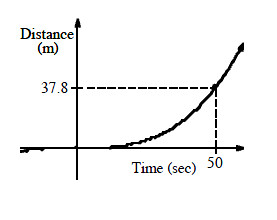2.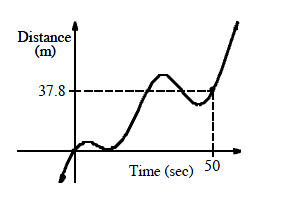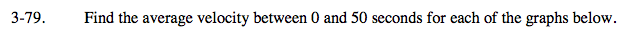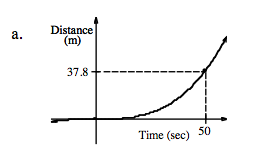$\text{Average velocity} = \frac{\Delta \text{distance}}{\Delta \text{time}}$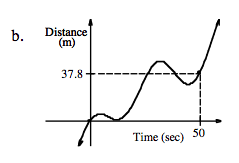This graph shows both forward and backwards motion. Will that affect average velocity?